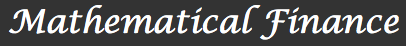Introduction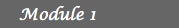1. The Binomial Model 2. Arbitrage opportunities 3. The First Fundamental  Theorem of Asset Pricing 4. Financial Derivatives 5. Hedging 6. Solutions7. Dividend payments 8. Families of risky assets 9. Time dependent interest  rates and rates of return 10. Short-selling prohibition 11. Multi-factor models 12. SolutionsFinancial Derivatives

In the previous lessons we introduced the Binomial Model. As we saw this model consists of one riskless asset whose price changes over time by a fixed interest rate r and one risky asset such that its rate of return at any time can only take two possible values a or b with probabilities p and 1-p, respectively. This rather simple structure prompted an interesting question about conditions for the existence of strategies to beat the market or arbitrage opportunities. Regarding this issue we presented the First Fundamental Theorem of Asset Pricing reaching the conclusion that if p is not 0 or 1 the market is arbitrage-free if and only if a < r < b .

In this section we will give a brief introduction to the concept of Financial Derivative. Financial Derivatives are financial instruments used by investors to reduce the risk in the market. These instruments give a more complex structure to Financial Markets and elicit one of the main problems in Mathematical Finance, namely to find fair prices for them. Under more complicated models this question can be very hard but under our binomial model is relatively easy to answer.

We say that y depends linearly on x1, x2, ..., xm if y=a1x1+a2x2+ ... + amxm for some constants a1, a2, ..., am. Hence, the payoff of a financial derivative is not of the form aS0+bS, with a and b constants.

Formally a Financial Derivative is a security whose payoff depends in a non-linear way on the primary assets, S0 and S in our model (see Tangent). They are also called derivative securities and are part of a broarder cathegory known as contingent claims. Contingent claims do not necessarily depend on the primary assets. There exists a large number of derivative securities that are traded in the market, below we present some of them.

## Forward Contract

Under a forward contract, one agent agrees to sell to another agent the risky asset at a future time for a price K which is specified at time 0. The owner of a Forward Contract on the risky asset S with maturity T gains the difference between the actual market price ST and the delivery price K if ST is larger than K at time T. If ST < k, the owner loses the amount K-ST to the issuer of the forward contract. Therefore, we can express the payoff of Forward Contract by## Call Option

The owner of a call option on the risky asset S has the right, but no the obligation, to buy the asset at a future time for a fixed price K, called strike price. When the owner has to exercise the option at maturity time the option is called a European Call Option. When the owner can choose the time to exercise his option it is known as an American Call Option. The payoff of a European Call Option is of the form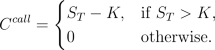## Put Option

Conversely, a put option gives the right, but no the obligation, to sell the asset at a future time for a fixed price K, called strike price. As before when the owner has to exercise the option at maturity time the option is called a European Put Option. When the owner can choose the time to exercise his option it is known as an American Put Option. The payoff of a European Put Option is of the form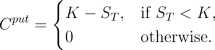## Remarks

We have seen in the previous examples that there are two categories of options, European type options and American type options. This extends also to financial derivatives in general. The difference between the two is that for European type derivatives the owner of the contract can only "exercise" at a fixed maturity time whereas for American type derivative the "exercise time" could occur before maturity. In this section we will concentrate our attention on European type derivatives because they are easier to price than American type derivatives.

There is a close relation between forwards and European call and put options which is expressed in the following equation known as the put-call parity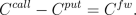Hence, the payoff at maturity from buying a forward contract is the same than the payoff from buying a European call option and short selling a European put option.

## Pricing

Once financial instruments like forwards and European call and put options are defined the first question to answer is: what is the fair price that the seller of a Financial Derivative should charge to the buyer? In the previous lesson we have developed the machinery necessary to answer this question. A fair price of a European Type Derivative is the expectation of the discounted final payoff with repect to a risk-neutral probability measure. These are fair prices because with them the extended market in which the derivatives are traded assets is arbitrage free (see the fundamental theorem of asset pricing).

In the arbitrage-free CRR model with p strictly between 0 and 1 and a < r < b according to Acitvity 2 in the previous lesson we obtain the following prices for forwards and European call and put options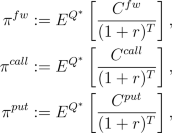where,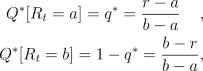for any time t. For instance, consider the market given in Example 3 but with r=0. In this case b=0.01 and a=-0.03. The risk neutral measure is given then byConsider a European call option with maturity of 2 days (T=2) and strike price K=10*(0.97). The risk neutral measure and possible payoffs of this call option can be included in the binary tree of the stock price as follows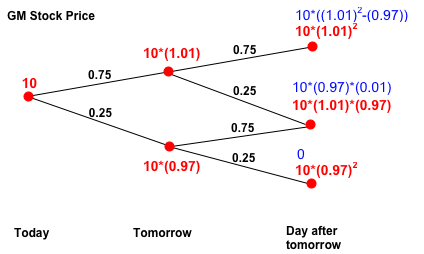We find then that the price of this European call option is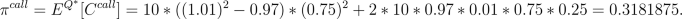It is easy to see that the price of a forward contract with the same maturity and same forward price K is given by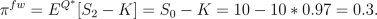By the put-call parity mentioned above we deduce that the price of an European put option with same maturity and same strike is given by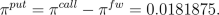That the call option is more expensive than the put option is due to the fact that in this market, the prices are more likely to go up than down under the risk-neutral probability measure. This does not happen if one considers the initial probabilities p and 1-p.

## Remark

Initially one is tempted to believe that for high values of p the price of the call option should be larger since it is more certain that the price of the stock will go up. However our arbitrage free argument leads to the same price for any probability p strictly between 0 and 1. How can this be? The best economical explanation is the following: If the probability p is almost one this reflects a change in the market participants risk aversion. Hence for large values of p either the whole price structure changes or the risk aversion of the participants change and they value less any potential gain and are more averse to any loss.

Activities
1. A straddle is a derivative whose payoff increases proportionally to the change of the price of the risky asset. Specifically, a straddle pays at time T the difference between the price ST and the price S0 if this difference is positive and minus the difference if it is negative. Basically with a straddle one is betting on the price move, regardless of the direction of this move. Write down explicitely the payoff of a straddle and find the price of a straddle with maturity T=2 for the model described above.
2. Suppose that you want to buy the text-book for your math finance class in two days. Today the price of the book is \$80 dollars. You know that each day the price of the book goes up by 20% and down by 10% with the same probability. Assume that you can borrow or lend money with no interest rate. The bookstore offers you the option to buy the book the day after tomorrow for \$80. What is the fair fee the bookstore should charge you for this option?
3. Consider the same situation than in b). Now the library offers you what is called a discount certificate, you will receive the smallest amount between the price of the book in two days and a fixed quantity, say \$80. What is the fair price of this contract?